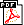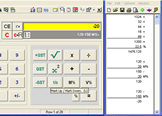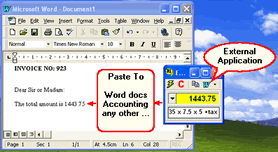RICalcFinancialInvestmentInflationGraphingStatisticsScientific / Other DownloadsFree demo Online shopBuy online AboutContact us LinksResources

 Take a Tour For Home For ProfessionalsBrochure
(328 KB)# Scientific calculator with Tape and Expression Evaluator

A calculator for Scientific, Standard and base conversions.

RICalc a scientific calculator with plenty of power.

Operators, memory and other buttons, work the same way as your regular pocket calculator.

## General calculator

RICalc calculator has the following modes of operation:Standard general + tax, markup, discount.Scientific calculus, trigonometry, stats.Hexadecimal base 16 and base 10 conversions.Octal base 8 and base 10 conversions.Binary base 2 and base 10 conversions. To work with external applications, zoom calculator (about 60%) or use EE.

## Tape calculatorCalculator with Tape

Print calculations on Tape and save it for retrieval when a long list of operations.
Change Tape values and operators and tape is automatically recalculated.Save word documents or store temporary
calculations on the built-in word notepad.

## Date calculatorDate periods calculator

Quick calculation of dates
US Federal calendar days
.

## Expression Evaluator

EE is an algebraic expression calculator.
A rapid calculator with one-click paste to external applications.
With EE you just type in the expression, it is faster than pressing buttons.Paste To external

One click Transfer To your
word processor, accounting and other external applications.

Expressions can be simple or quite complex mathematical formulas
Either way the Expression Evaluator is easier and faster to work with than pocket calculators.

## Multi precision

RICalc has an internal multi-precision engine capable of performing calculations to an arbitrary precision of millions of digits of accuracy.

Multi precision is used in RICalc for calculation of mathematical constants, such as pi, e, euler to high precision, and to improve accuracy in functions such as factorials, gamma, beta, root finder, integrals, trigonometry, polynomial, complex and other functions.

Most multi-precision implementations are already available in EE and in Functions.

Calculate algebraic expressions to high-precision.

## Functions

400+ predefined functions for:

Math, Trigonometry, Statistic, Financial, Conversion, Logical, Text, Date, Information, Geometry, and more...

Create and store your own functions.

Functions prefixed with MP, perform arithmetic computation in arbitrary precision.

Non MP functions with precision parameter in the argument list, compute in either IEEE or in MP.

Select select MP format in the Expression Evaluator and most expressions are performed in high-precision.

How can you use the Scientific calculator and Expression Evaluator?
For rapid calculations, use the Expression Evaluator and one-click paste to external applications.
For general calculations, use the General - Scientific calculator.
For multi precision and to solve hundreds of functions, use Functions.
For Time, use the Date calculator and the others tools for quick and easy calculations.

Clear and easy calculators for what mattersSave time

All-in-one true calculator, not several, just one.

Scientific calculator with Tape / Expression calculator / Date / Functions high precision® Product and company names are trademarks or registered trademarksPrivacyDisclaimerSite Map © 2020 DBCOM software